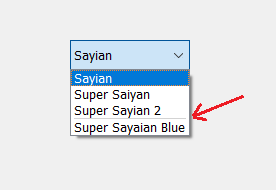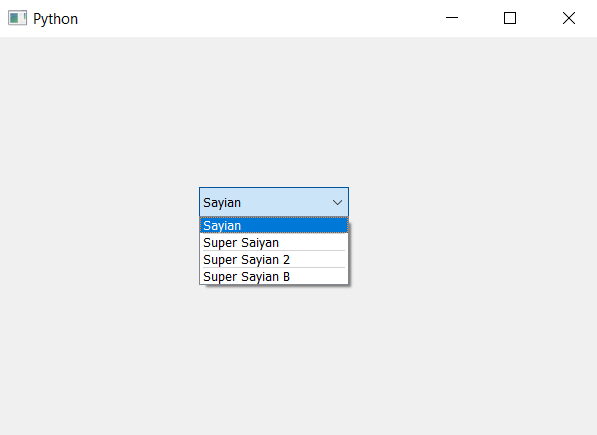# PyQt5 – Add multiple separator in between item of ComboBox

In this article we will see how we can add multiple of separator in between the items of the combo box, by default there is no separator. Separator is the black line in between two items, in order to add he separator we use `insertSeparator` method. Below is representation of how combo box items with a separator looks likeIn order to add multiple separator we will use `insertSeparator` method multiple times but initial giving the index value of max index then second maximum index value and so on, if we first give the minimum index value then other statements will only increse the size of the minimum index vale separator.

For adding separator at two places only
Syntax :
combo_box.insertSeparator(max(indexs))
combo_box.insertSeparator(min(indexs)

Here, indexes is the list of two index

Argument : It takes integer as argument i.e index

Action performed : It will add separator at the given index

Below is the implementation –

 `# importing libraries ` `from` `PyQt5.QtWidgets ``import` `*`  `from` `PyQt5 ``import` `QtCore, QtGui ` `from` `PyQt5.QtGui ``import` `*`  `from` `PyQt5.QtCore ``import` `*`  `import` `sys ` ` `  ` `  `class` `Window(QMainWindow): ` ` `  `    ``def` `__init__(``self``): ` `        ``super``().__init__() ` ` `  `        ``# setting title ` `        ``self``.setWindowTitle(``"Python "``) ` ` `  `        ``# setting geometry ` `        ``self``.setGeometry(``100``, ``100``, ``600``, ``400``) ` ` `  `        ``# calling method ` `        ``self``.UiComponents() ` ` `  `        ``# showing all the widgets ` `        ``self``.show() ` ` `  `    ``# method for widgets ` `    ``def` `UiComponents(``self``): ` ` `  `        ``# creating a combo box widget ` `        ``self``.combo_box ``=` `QComboBox(``self``) ` ` `  `        ``# setting geometry of combo box ` `        ``self``.combo_box.setGeometry(``200``, ``150``, ``150``, ``30``) ` ` `  `        ``# geek list ` `        ``geek_list ``=` `[``"Sayian"``, ``"Super Saiyan"``, ``"Super Sayian 2"``, ``"Super Sayian B"``] ` ` `  `        ``# adding list of items to combo box ` `        ``self``.combo_box.addItems(geek_list) ` ` `  `        ``# index list ` `        ``indexs ``=` `[``1``, ``3``, ``2``] ` ` `  `        ``# adding separator at maximum index ` `        ``self``.combo_box.insertSeparator(``max``(indexs)) ` ` `  `        ``# adding separator at middle index ` `        ``index ``=` `0` `        ``for` `i ``in` `indexs: ` `            ``if` `i > ``min``(indexs) ``and` `i < ``max``(indexs): ` `                ``index ``=` `i ` `        ``self``.combo_box.insertSeparator(index) ` ` `  `        ``# adding separator at minimum index ` `        ``self``.combo_box.insertSeparator(``min``(indexs)) ` ` `  ` `  `# create pyqt5 app ` `App ``=` `QApplication(sys.argv) ` ` `  `# create the instance of our Window ` `window ``=` `Window() ` ` `  `# start the app ` `sys.exit(App.``exec``()) `

Output :My Personal Notes arrow_drop_upCheck out this Author's contributed articles.

If you like GeeksforGeeks and would like to contribute, you can also write an article using contribute.geeksforgeeks.org or mail your article to contribute@geeksforgeeks.org. See your article appearing on the GeeksforGeeks main page and help other Geeks.

Please Improve this article if you find anything incorrect by clicking on the "Improve Article" button below.

Article Tags :

Be the First to upvote.

Please write to us at contribute@geeksforgeeks.org to report any issue with the above content.